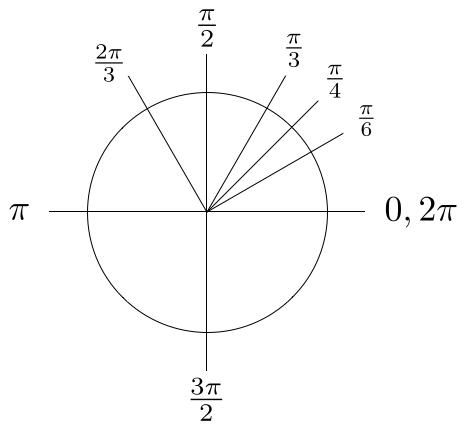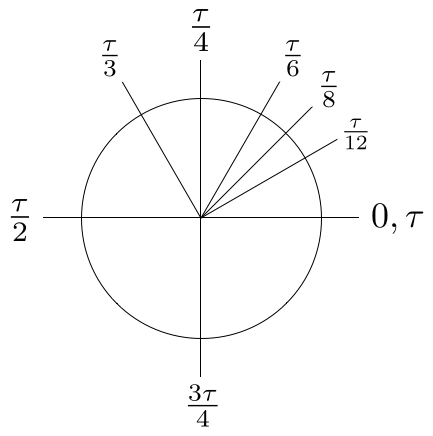# down with pi, long live tau

An article at CNN on this most mathy of mornings (it's pi day, today), points to a manifesto written by a Michael Hartl, a mathematician who is attempting to get the circle constant redefined away from π (pi - the ratio of a circle's circumference to its diameter). He'd like to replace it with τ (tau), which he uses to represent the ratio of a circle's circumference to its radius, which is 2π.

While those who have advanced beyond basic trigonometry and geometry might say, "What's the big deal? How hard is it to divide and multiply by two?" I think that he makes a compelling argument. Using τ to teach geometry and trig makes the underlying mathematical principals clearer and easier to understand. Take a look at the following two graphs, which Hartl uses to illustrate his point.Special angles of a circle, in π units.Special angles of a circle, in τ units.

The difference in those two graphs is enough to convert me, but he points out several other concepts that are clarified by using τ: the circle functions of sin and cos, and complex exponentiation, to name a few. At the very least, it makes explaining these concepts far simpler. It's certainly something I'm going to keep in mind for the future.

### David Garrett

One day, I will have a super-secret moon base and an army of ninja. Until then, I manage software engineers.